# X-Y CoordinatesBasics
X-Y Coordinate Plane
• flat 2-dimensional field for plotting points anywhere along x-y axes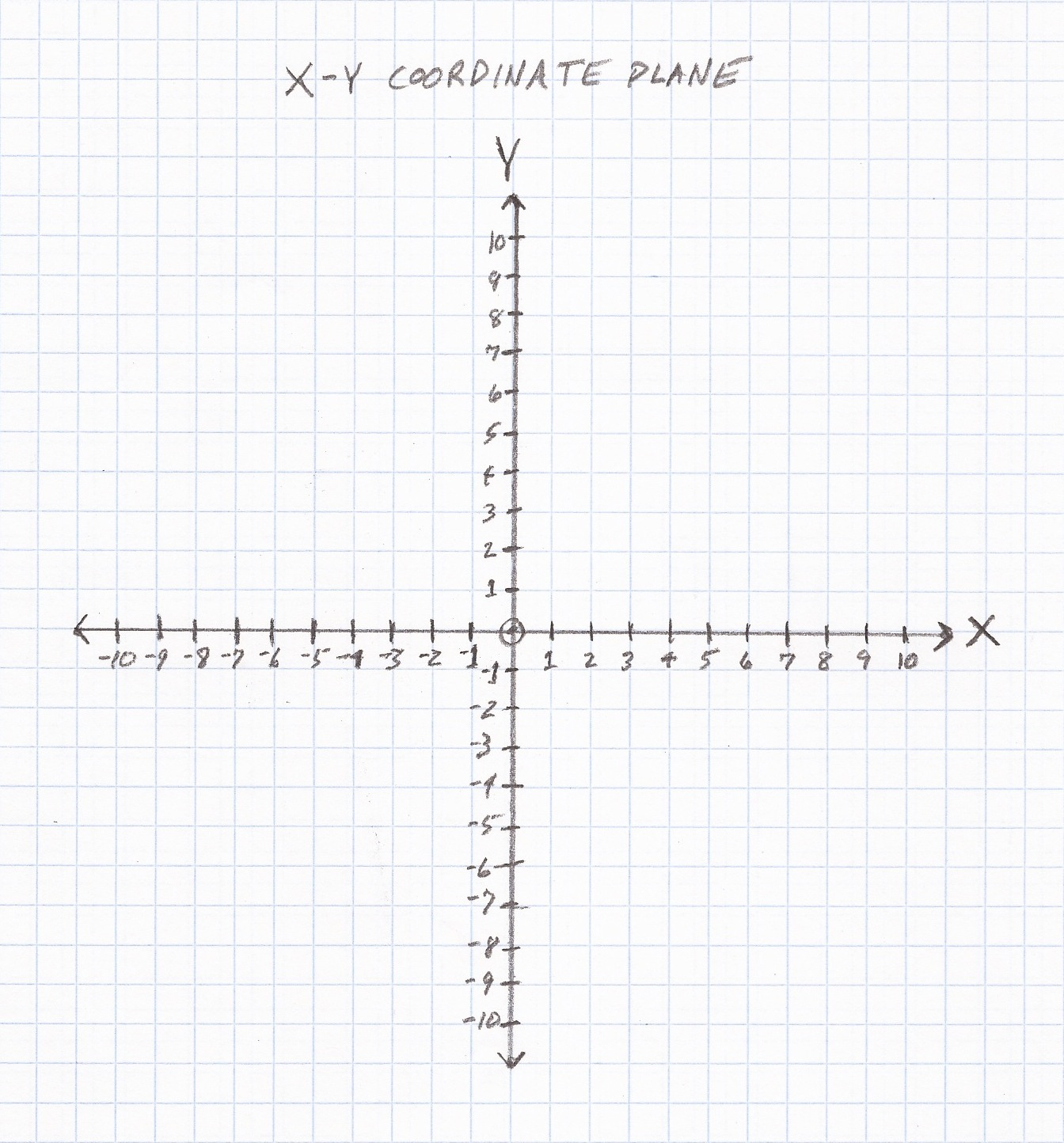X-Y Coordinate Grid
• flat 2-dimensional field limited to plotting positive points along x-y axes
• x-axis = horizontal dimension, extending infinitely
• y-axis – vertical dimension, extending infinitely
• A point is plotted according to its position (x, y) along the x-y axes.Question

Plot (0, 0) on the x-y coordinate plane.Question

Plot (0, 0) on the x-y coordinate grid.Question

Plot (5, 3) on the x-y coordinate plane.• From (0, 0), move 5 spaces to the right along the positive x-axis.
• Then move 3 spaces up parallel with the positive y-axis.
• Arrive at point (5, 3).

Question

Plot (5, 3) on the x-y coordinate grid.• From (0, 0), move 5 spaces to the right along the positive x-axis.
• Then move 3 spaces up parallel with the positive y-axis.
• Arrive at point (5, 3).

Question

Plot (5, -3) on the x-y coordinate plane.• From (0, 0), move 5 spaces to the right along the positive x-axis.
• Then move 3 spaces down parallel with the negative y-axis.
• Arrive at point (5, -3).

Question

Plot (5, -3) on the x-y coordinate grid.Because the x-y coordinate grid requires both x and y to be positive numbers, there is no place to plot (5, -3).

Question

Plot (-5, 3) on the x-y coordinate plane.• From (0, 0), move 5 spaces to the left along the negative x-axis.
• Then move 3 spaces up parallel with the positive y-axis.
• Arrive at point (-5, 3).

Question

Plot (-5, 3) on the x-y coordinate grid.Because the x-y coordinate grid requires both x and y to be positive numbers, there is no place to plot (-5, 3).

Question
Plot (-5, -3) on the x-y coordinate plane.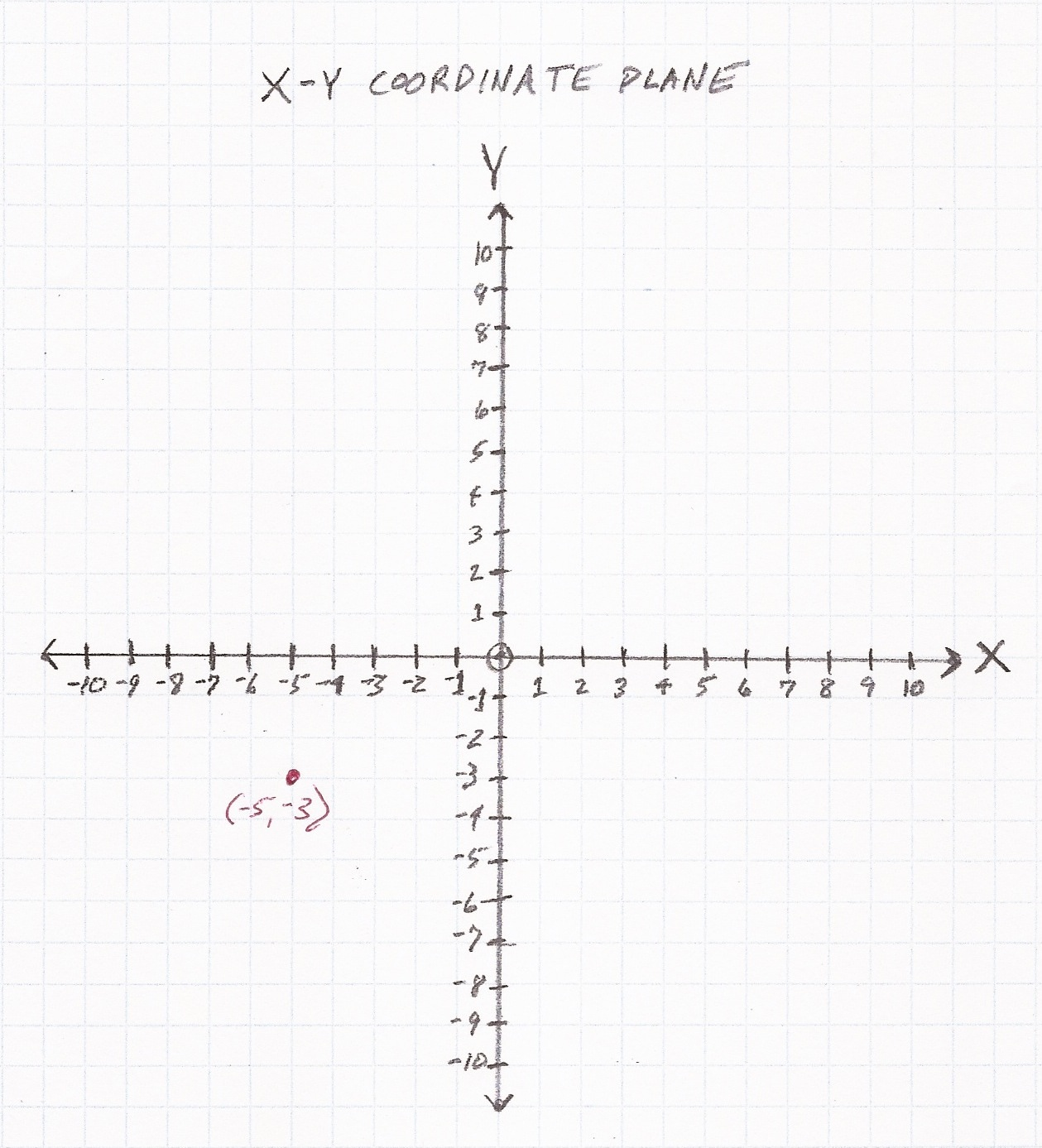• From (0, 0), move 5 spaces to the left along the negative x-axis.
• Then move 3 spaces down parallel with the negative y-axis.
• Arrive at point (-5, -3).

Question

Plot (-5, -3) on the x-y coordinate grid.Because the x-y coordinate grid requires both x and y to be positive numbers, there is no place to plot (-5, -3).

Question
The following table shows the age in years and height in inches of a boy and a girl.  On the accompanying x-y coordinate grid, plot the data for the boy at age 4 and at age 7.

 Boy Girl Age (yr) Height (in) Age (yr) Height (in) 2 34.00 2 33.50 3 37.50 3 37.00 4 40.00 4 39.50 5 43.00 5 42.25 6 45.25 6 45.00 7 48.00 7 48.00 8 50.25 8 50.00 9 52.50 9 52.50 10 54.50 10 54.25• From (0, 0), move to age 4 along the positive x-axis.
• Then move to height 40.00 parallel with the positive y-axis.
• Arrive at point (4, 40.00).

• From (0, 0), move to age 7 along the positive x-axis.
• Then move to approximate height 48.00 parallel with the positive y-axis.
• Arrive at point (7, 48.00).

Question

The following graph shows the level of the Dow Jones Industrial Average (stock market) at the beginning of each year from 2000 to 2005.  Based on the graph, the Dow Jones Industrial Average decreased in the years:
A.  2003 – 2004
B.  2004 – 2005
C.  2003 – 2005
D.  2000 – 2003
E.  2000 – 2000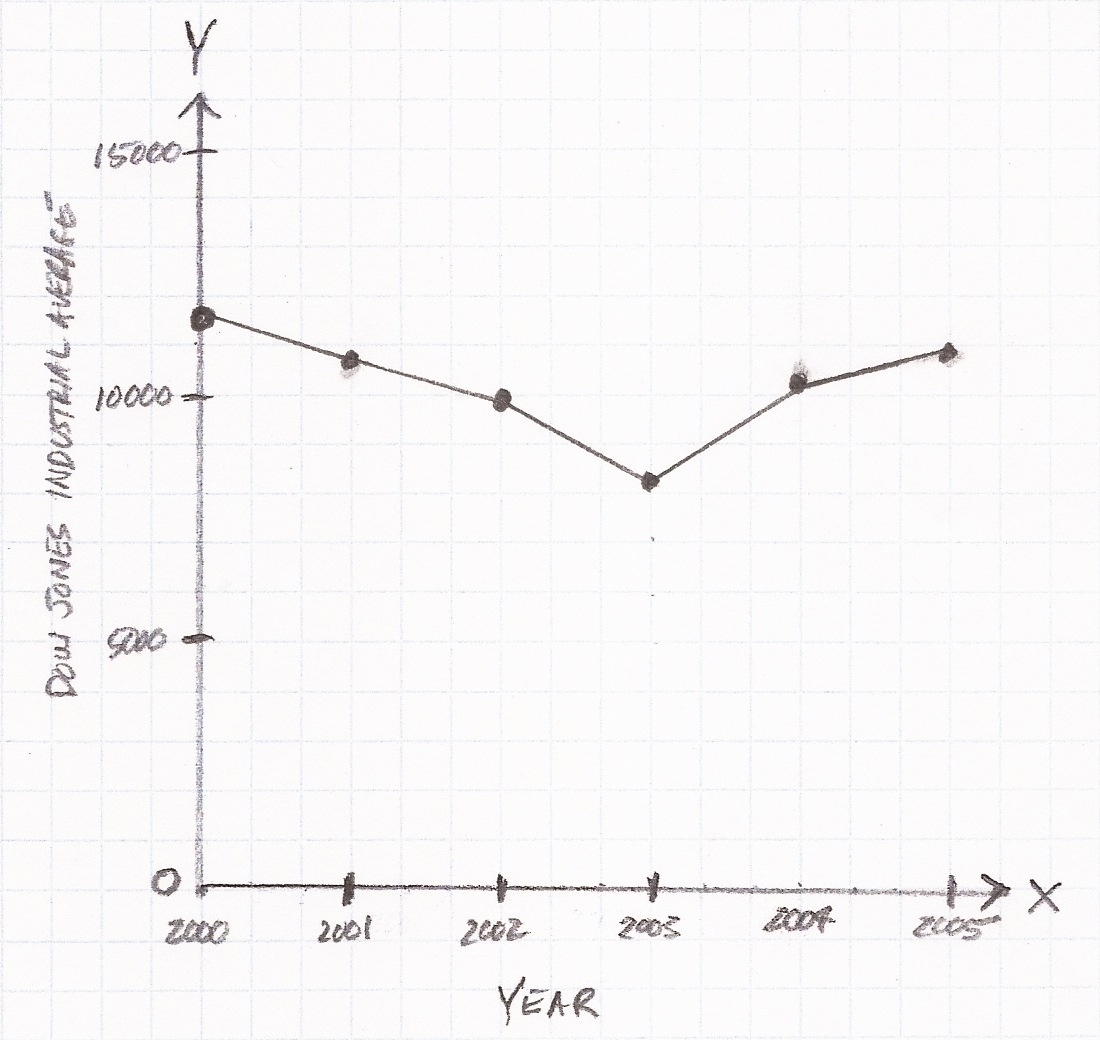D.  2000 – 2003

Based on the graph, which is an x-y coordinate grid, the Dow Jones Industrial Average decreased from (2000, 11500) to (2003, 8300) before increasing in subsequent years.

Practice Questions

1.  Plot (-6, 2) on the x-y coordinate plane.2.  Plot (-6, -2) on the x-y coordinate plane.3.  Plot (6, -2) on the x-y coordinate plane.4.  Plot (6, 2) on the x-y coordinate grid.5.  The following table shows the age in years and height in inches of a boy and a girl.  On the accompanying x-y coordinate grid, plot the data for the boy at age 2 and at age 5.

 Boy Girl Age (yr) Height (in) Age (yr) Height (in) 2 34.00 2 33.50 3 37.50 3 37.00 4 40.00 4 39.50 5 43.00 5 42.25 6 45.25 6 45.00 7 48.00 7 48.00 8 50.25 8 50.00 9 52.50 9 52.50 10 54.50 10 54.256.  With reference to the table and grid in Question 3, plot the data for the girl at age 3 and at age 6.

7.  With reference to the table and grid in Question 3, plot the data for the girl at age 7 and at age 8.

8.  The following graph shows the level of the Dow Jones Industrial Average (stock market) at the beginning of each year from 2000 to 2005.  Based on the graph, the Dow Jones Industrial Average increased in the years:
A.  2000 – 2001
B.  2001 – 2002
C.  2002 – 2003
D.  2003 – 2005
E.  2000 – 20039.  The following table shows the approximate weight in kilograms (kg) over the first ten weeks of a pig’s life.  On the accompanying x-y coordinate grid, plot the data for the pig at week 5 and at week 8.

 Age (weeks) Weight (kg) 0 1 1 2 2 3 3 5 4 8 5 10 6 15 7 18 8 20 9 25 10 3010.  The following graph shows the population of a city every ten years from 1900 through 2000.  Based on the graph, the population increased in the years:
A.  1960 – 1970
B.  1970 – 1980
C.  1900 – 1950
D.  1980 – 1990
E.  1950 – 20001.2.3.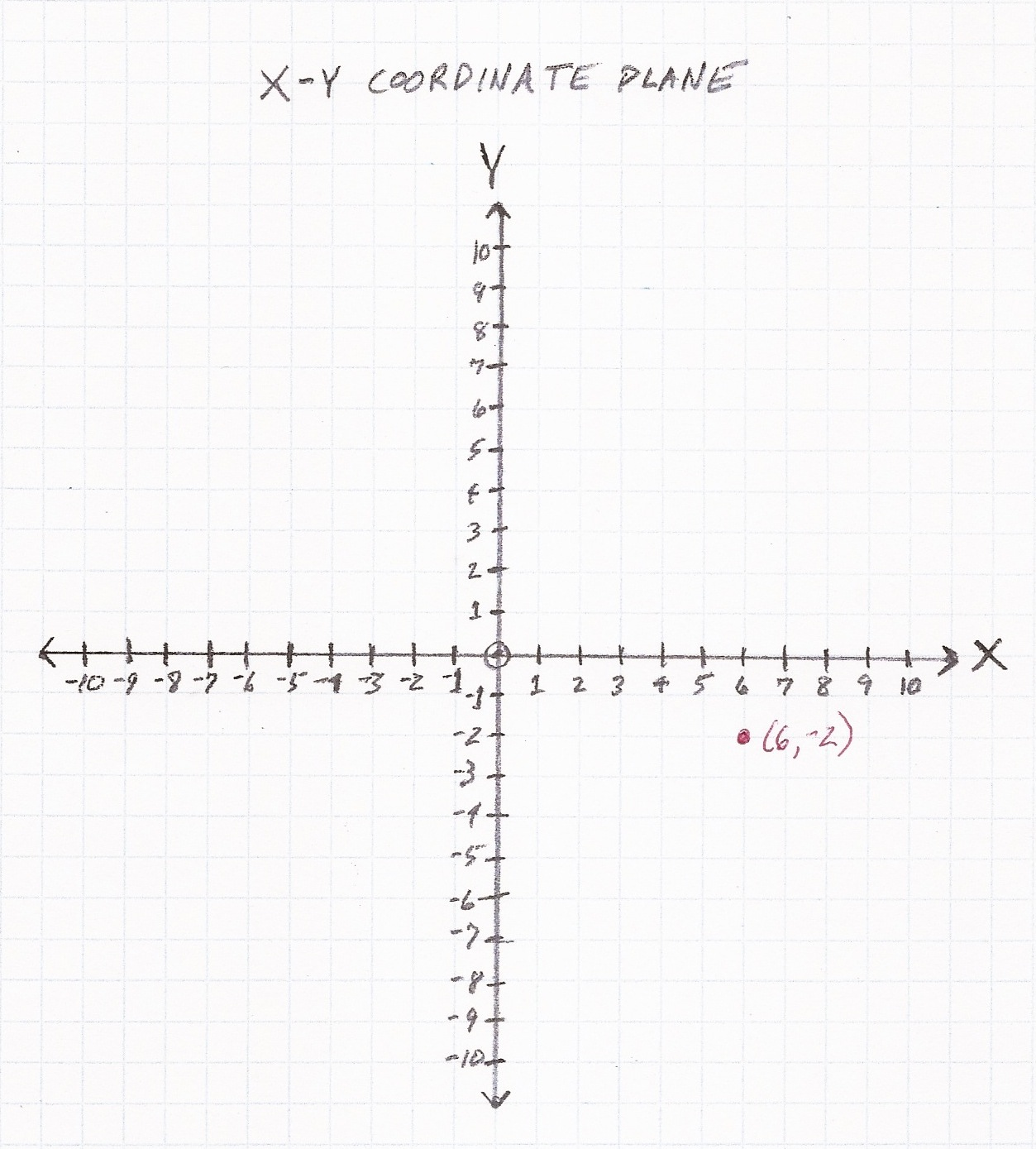4.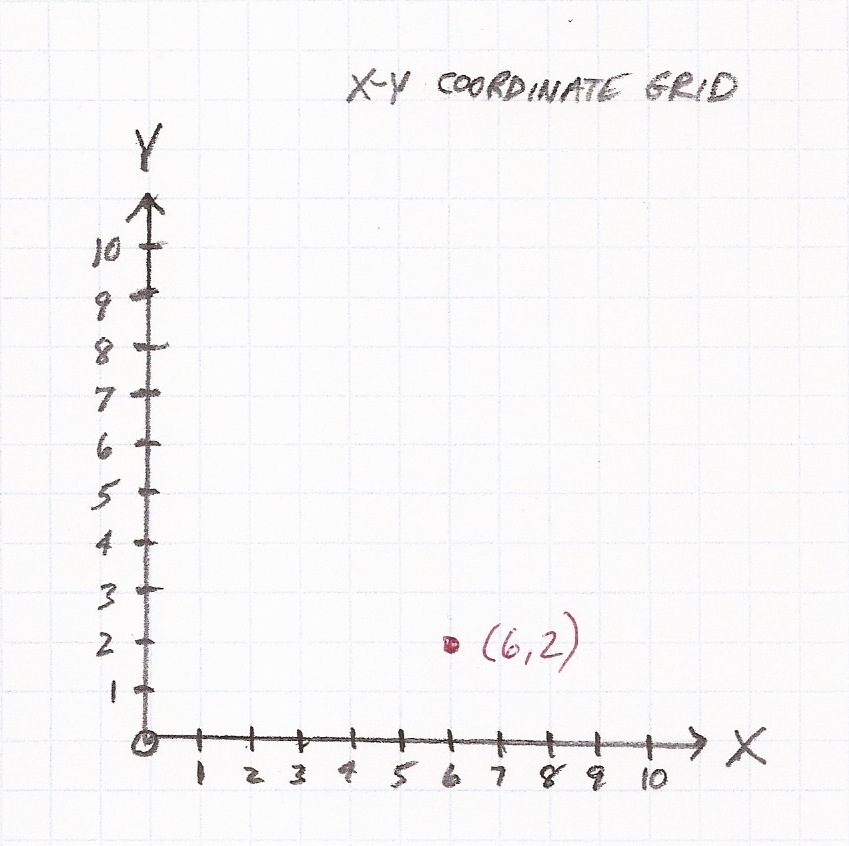5.6.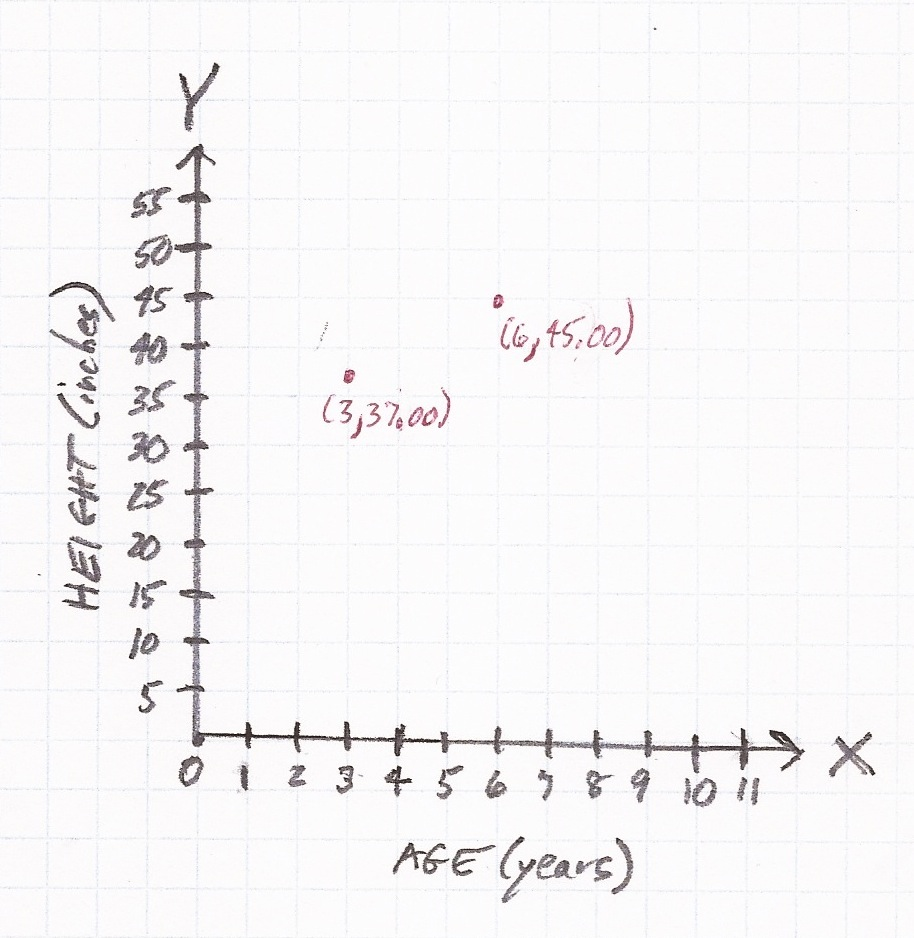7.8.  D.  2003 – 2005

9.10.  C.  1900 – 1950